## RS Aggarwal Class 10 Solutions Chapter 10 Quadratic Equations Ex 10B

These Solutions are part of RS Aggarwal Solutions Class 10. Here we have given RS Aggarwal Solutions Class 10 Chapter 10 Quadratic Equations Ex 10B.

Other Exercises

Solve each of the following equations by using the method of completing the square:
Question 1.
Solution:
x² – 6x + 3 = 0
=> x² – 2 x 3 x x = -3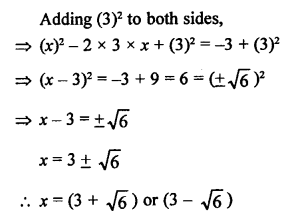Question 2.
Solution: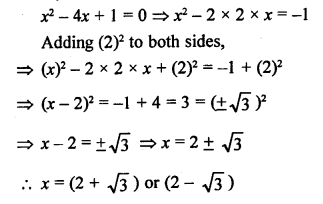Question 3.
Solution: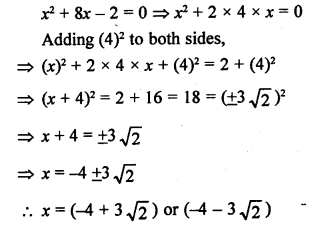Question 4.
Solution: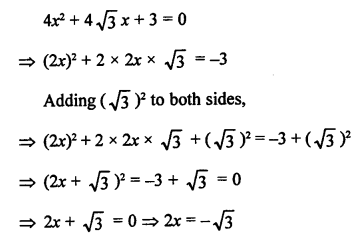Question 5.
Solution: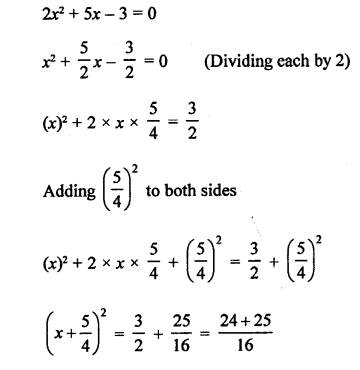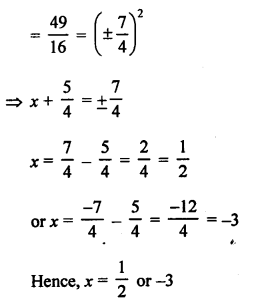Question 6.
Solution: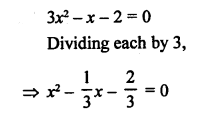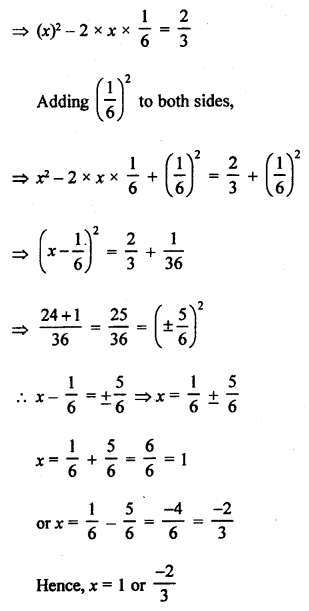Question 7.
Solution: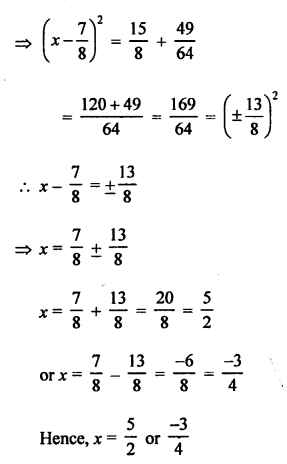Question 8.
Solution: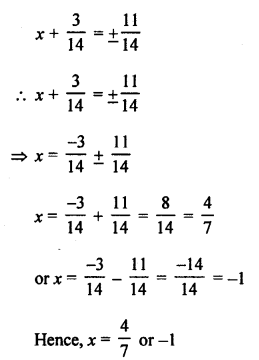Question 9.
Solution: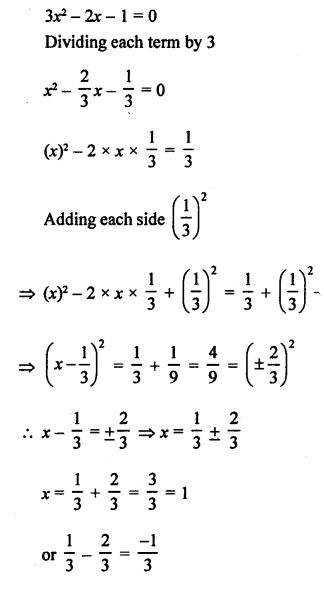Question 10.
Solution:Question 11.
Solution: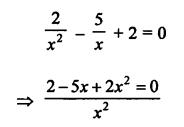Question 12.
Solution: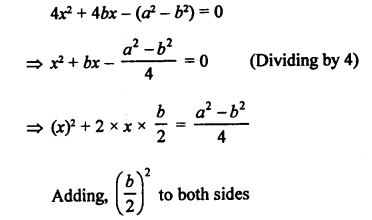Question 13.
Solution: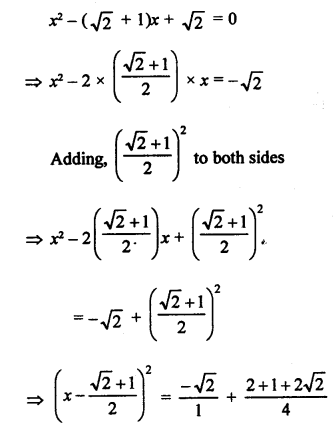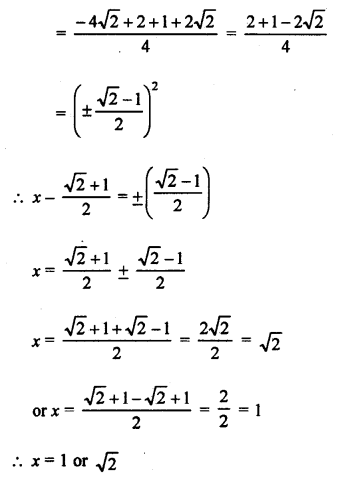Question 14.
Solution: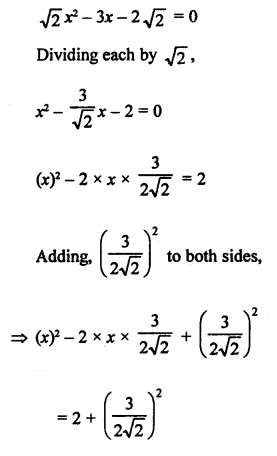Question 15.
Solution: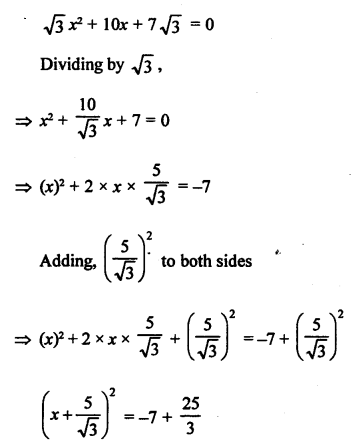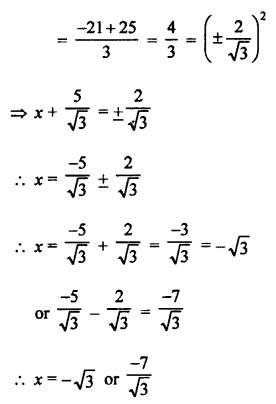Question 16.
Solution:Hope given RS Aggarwal Solutions Class 10 Chapter 10 Quadratic Equations Ex 10B are helpful to complete your math homework.

If you have any doubts, please comment below. Learn Insta try to provide online math tutoring for you.